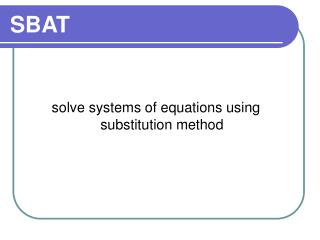# SBAT - PowerPoint PPT PresentationDownload PresentationSBAT

SBATDownload Presentation## SBAT

- - - - - - - - - - - - - - - - - - - - - - - - - - - E N D - - - - - - - - - - - - - - - - - - - - - - - - - - -
##### Presentation Transcript

1. SBAT solve systems of equations using substitution method

2. Solving Systems of Equations • You can solve a system of equations using different methods. The idea is to determine which method is easiest for that particular problem. • This document shows how to solve systems of equations algebraically using SUBSTITUTION.

3. Solving a system of equations by substitution Pick the easier equation. The goal is to get y= ; x= ; a= ; etc. Step 1: Solve an equation for one variable. Step 2: Substitute Put the equation solved in Step 1 into the other equation. Step 3: Solve the equation. Get the variable by itself. Step 4: Plug back in to find the other variable. Substitute the value of the variable into the equation. Step 5: Check your solution. Substitute your ordered pair into BOTH equations.

4. 1) Solve the system using substitution x + y = 5 y = 3 + x Step 1: Solve an equation for one variable. The second equation is already solved for y! Step 2: Substitute x + y = 5x + (3 + x) = 5 2x + 3 = 5 2x = 2 x = 1 Step 3: Solve the equation.

5. 1) Solve the system using substitution x + y = 5 y = 3 + x x + y = 5 (1) + y = 5 y = 4 Step 4: Plug back in to find the other variable. (1, 4) (1) + (4) = 5 (4) = 3 + (1) Step 5: Check your solution. The solution is (1, 4). What do you think the answer would be if you graphed the two equations?

6. Which answer checks correctly? • (2, 2) • (5, 3) • (3, 5) • (3, -5) 3x – y = 4 x = 4y - 17

7. 2) Solve the system using substitution 3y + x = 7 4x – 2y = 0 It is easiest to solve the first equation for x. 3y + x = 7 -3y -3y x = -3y + 7 Step 1: Solve an equation for one variable. Step 2: Substitute 4x – 2y = 0 4(-3y + 7) – 2y = 0

8. 2) Solve the system using substitution 3y + x = 7 4x – 2y = 0 -12y + 28 – 2y = 0 -14y + 28 = 0 -14y = -28 y = 2 Step 3: Solve the equation. 4x – 2y = 0 4x – 2(2) = 0 4x – 4 = 0 4x = 4 x = 1 Step 4: Plug back in to find the other variable.

9. 2) Solve the system using substitution 3y + x = 7 4x – 2y = 0 Step 5: Check your solution. (1, 2) 3(2) + (1) = 7 4(1) – 2(2) = 0 When is solving systems by substitution easier to do than graphing? When only one of the equations has a variable already isolated (like in example #1).

10. If you solved the first equation for x, what would be substituted into the bottom equation. • -4y + 4 • -2y + 2 • -2x + 4 • -2y+ 22 2x + 4y = 4 3x + 2y = 22

11. 3) Solve the system using substitution x = 3 – y x + y = 7 Step 1: Solve an equation for one variable. The first equation is already solved for x! Step 2: Substitute x + y = 7 (3 – y) + y = 7 3 = 7 The variables were eliminated!! This is a special case. Does 3 = 7? FALSE! Step 3: Solve the equation. When the result is FALSE, the answer is NO SOLUTIONS.

12. 3) Solve the system using substitution 2x + y = 4 4x + 2y = 8 Step 1: Solve an equation for one variable. The first equation is easiest to solved for y! y = -2x + 4 4x + 2y = 8 4x + 2(-2x + 4) = 8 Step 2: Substitute 4x – 4x + 8 = 8 8 = 8 This is also a special case. Does 8 = 8? TRUE! Step 3: Solve the equation. When the result is TRUE, the answer is INFINITELY MANY SOLUTIONS.

13. What does it mean if the result is “TRUE”? • The lines intersect • The lines are parallel • The lines are coinciding • The lines reciprocate • I can spell my name